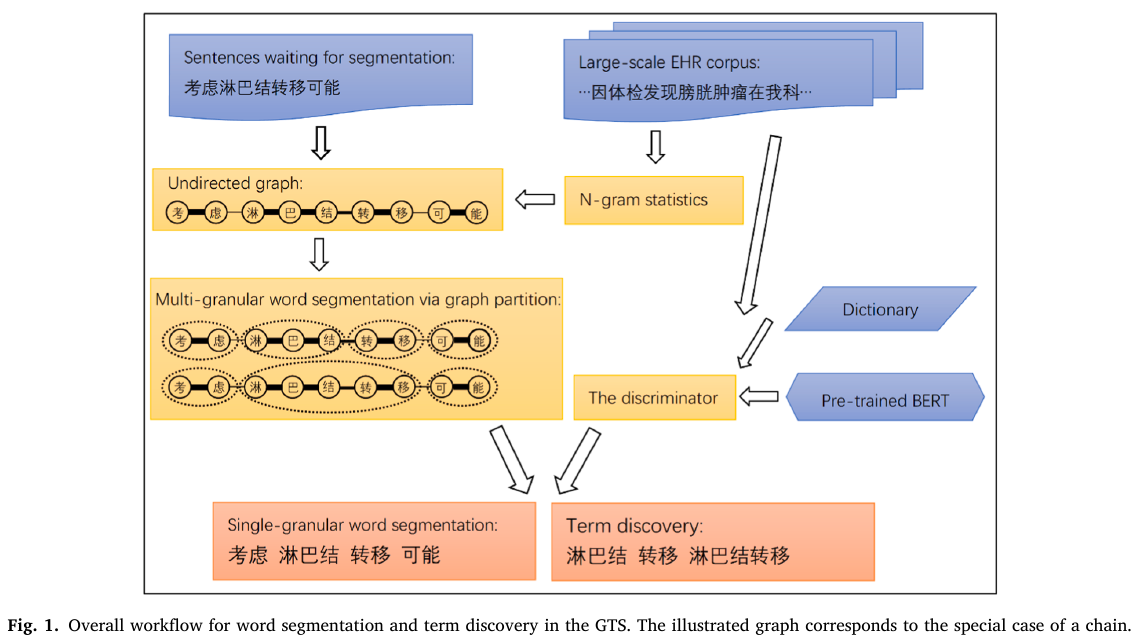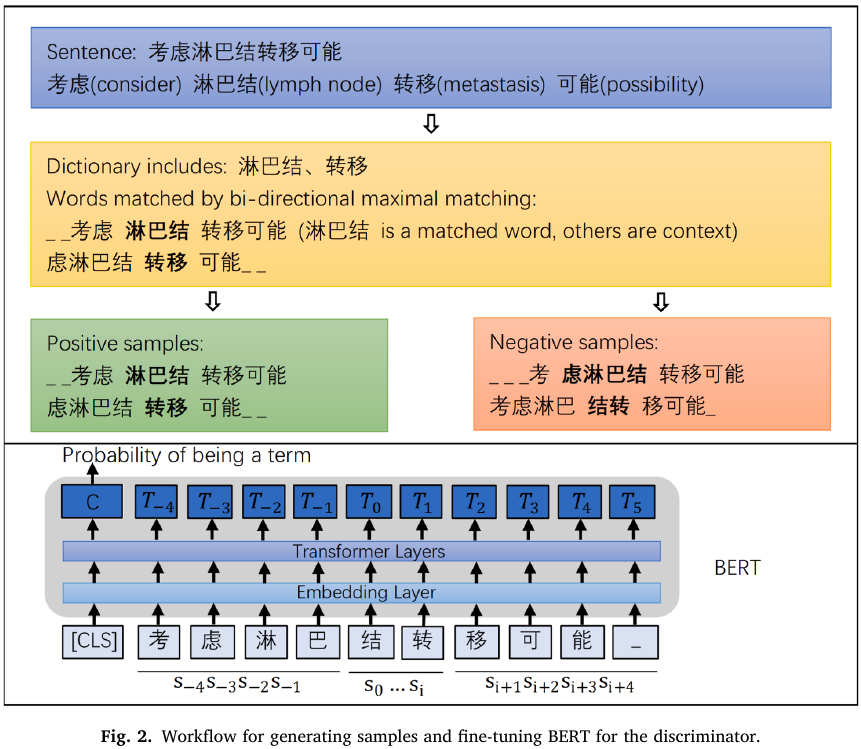# Unsupervised Multi-granular Chinese Word Segmentation via Graph Partition

##### Posted on Jun 03, 20210 Comments

Objective: unsupervised term discovery in Chinese electronic health records (EHRs) by using the word segmentation technique. - the existing supervised algorithms do not perform well in the case of EHRs, as annotated medical data are scarce. - the paper proposes an unsupervised segmentation method (GTS) based on the graph partition principle, whose multi-granular segmentation capability can help realize efficient term discovery.

Methods: - convert a sentence to an undirected graph, with the edge weights based on n-gram statistics, - use ratio cut to split the sentence into words - solve the graph partition via dynamic programming - realize multi-granularity by setting different partition numbers - train a BERT-based discriminator using generated samples to verify the correctness of the word boundaries. - the words that are not recorded in existing dictionaries are retained as potential medical terms

Results: - compare the GTS approach with mature segmentation systems for both word segmentation and term discovery. - MD students manually segmented Chinese EHRs at fine and coarse granularity levels and reviewed the term discovery results. - the proposed unsupervised method outperformed others

Conclusion: - the graph partition technique can effectively use the corpus statistics and even expert knowledge to realize unsupervised word segmentation of EHRs - multi-granular segmentation can be used to provide potential medical terms of various lengths with high accuracy.

## Introduction

Popular methods in the natural language processing in recent years: - distributed semantics: assigns numeric vector representations to words - end-to-end algorithms: map raw text inputs directly to final results

but concept extraction, which structures free text data by identifying mentions of predefined concepts, is still of fundamental importance to most down-stream algorithms and studies that require interpretability and interoperability.

Many commonly used concept extraction software for English, such as - MetaMap - cTAKES - CLAMP assume the unified medical language system (UMLS) as the default lexicon.

Open-ended concept extraction: - the most popular machine learning treatment is to formulate it as a sequential tagging problem, with different labels indicating whether a token is the beginning of the term, within the term, or not part of the term.

Word segmentation: - commonly required when performing the NLP of many East Asian languages, such as Chinese and Japanese, which do not use spaces as word boundaries in writing. - it does not entirely equal the concept of tokenization for English, e.g., word segmentation normally treats “类风湿性关节炎” (类风湿性=rheuma­toid, 关节炎=arthritis) as a single word, even though it consists of two subwords (which, in fact, can be further decomposed; e.g., 关节=joint, 炎=inflammation). - words in these languages can be multiword phrases in English, and the function of word segmentation can be considered to be include both tokenization and concept extraction/named entity recognition.

Existing word segmentation algorithms: - quite similar to concept extraction algorithms - dictionary-based algorithms, most of which are based on the maximal matching principle

Propose: - an unsupervised word segmentation method for term discovery based on graph theory and deep learning (referred to as graph term segmentation or GTS)

## Methodstwo parts: - segmenter: multi-granular word segmentation - discriminator: evaluate how likely it is for a piece of segmented string to be a term

### segmenter

• an undirected graph $G=(V,E)$, whose nodes $V={v_1,\ldots,v_n}$ represent the $n$ characters of a given sentence $s_1s_2\ldots s_n$.
• an $n\times n$ adjacent matrix $W$, where $w_{ij} (w_{ij}\ge 0\forall i,j)$ is the edge weight between the nodes $v_i$ and $v_j$

objective: group the nodes to $k$ mutually disjoint components $A_1,\ldots,A_k$ such that the edges crossing the components have low weights compared to the edges within the components

how to keep the sequence order?

Ohhh, just one line graph.

propose a design of $W$ based on the $n$-gram statistics

ratio cut and normalized cut are NP-hard problems, and can be solved approximately via an unsupervised method spectral clustering.

### discriminator

access how likely a string is a term, given its surrounding charactersPublished in categories Note